# BODE PLOTS单击或点击下面的示例电路以调用TINACloud并选择交互式DC模式以在线分析它们。or½K/s ½= 1。…以及截止频率： w1 = R / L = 5 / 0.5 = 10 rad / s f1 = 1.5916 Hz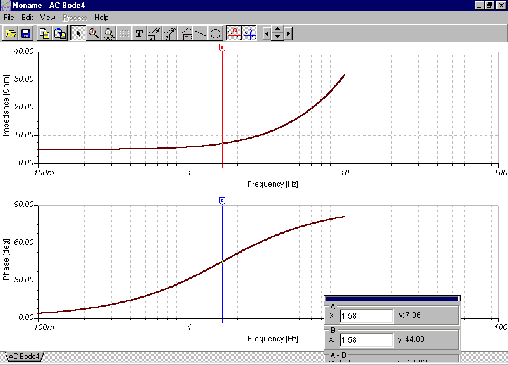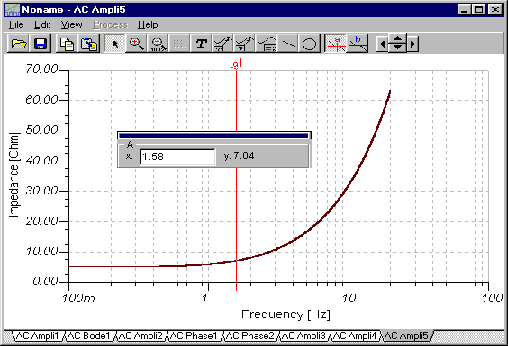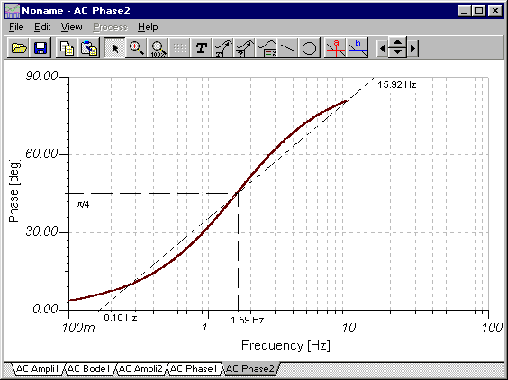# 例如2TINA的主要功能之一是其符号分析：分析–“符号分析” – AC传递或半符号AC传递。 这些分析以完全符号形式或半符号形式为您提供了网络的传递函数。 在半符号形式中，使用了组件值的数值，唯一剩余的变量是s。

TINA绘制实际的Bode图，而不是直线近似值。 要找到实际的截止频率，请使用光标找到–3 dB点。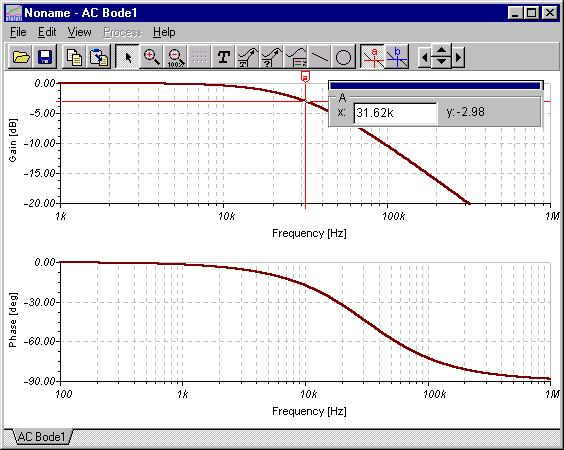y轴再次是线性的，并显示以dB为单位的电压比或以度为单位的相位。 x-或 w-轴表示以Hz为单位的频率。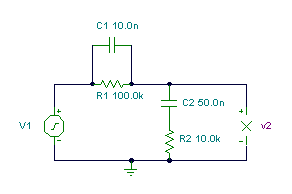单击/点击上面的电路以在线分析，或单击此链接以在Windows下保存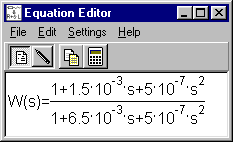w01 = 1 /（R1C1）= 103 弧度/秒 w02 = 1 /（R2C2）= 2 * 103 弧度/秒

f01 = 159.16 Hz f02 = 318.32 Hz

fP1 = 24.78 Hz fP2 = 2.044 kHzfP1 = 24.78 Hz

f01 = 159.16 Hz

f02 = 318.32 Hz

fP2 = 2.044 kHz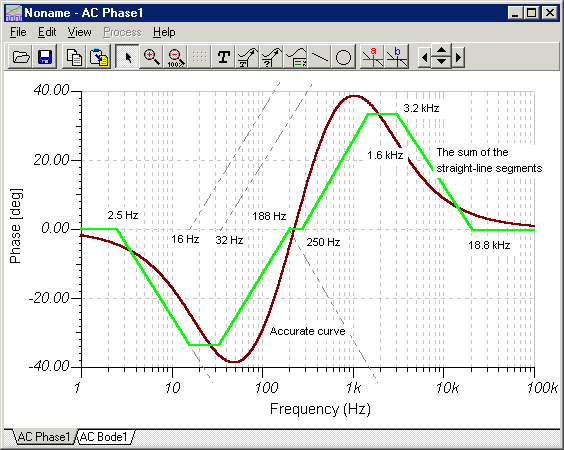A分钟 @ -12.74分贝 ® A分钟 = 0.23 at f = 227.7 Hz

j = fNNXX时的0 = 223.4。

X欢迎来到 DesignSoft
如果需要任何帮助找到合适的产品或需要支持，可以进行聊天。